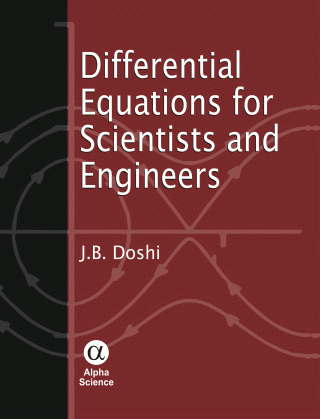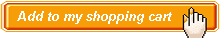Differential Equations for Scientists and Engineers Authors:   J. B. Doshi ISBN: 978-81-7319-789-5 Publication Year:   Reprint 2013 Pages:   342 Binding:   Paper Back About the book Differential Equations for Scientists and Engineers proposes to give a comprehensive treatment of differential equations encountered by scientists and engineers in various problems during their professional career. The book mainly deals with linear equations amenable to the so called analytical or exact solutions (including series solutions). However, exactly solvable nonlinear equations are covered briefly in the chapter on ordinary differential equations. A couple of chapters are included to deal with semi-analytical techniques, such as variational and other methods to deal with equations not solvable analytically. A chapter on nonlinear equations with modern treatment of first and second order systems including the concepts of bifurcations and limit cycles is included at the end. More advanced topics such as, the quasi-periodic motion and chaos are not included in the book. Throughout the book the emphasis is on the development and applications of solution techniques and the theoretical aspects regarding the existence and uniqueness of solutions are avoided or very briefly discussed with short proofs without disturbing the flow of the text. Key Features • Large Number of Solved Examples to Illustrate Solution Methods • Number of Problems in the Exercises at the End of Each Chapter Table of content Preface / Ordinary Differential Equations / Partial Differential Equations of First Order / Partial Differential Equations of Second Order / Basic Concepts in Approximate Solution to the Boundary Value Problems / Fourier Series / Hyperbolic Equation (Wave Equation) / Elliptic Equation (Potential Equation) / Parabolic Equation (Heat Equation) / Fourier Transforms / Variational Method and Other Approximate Methods / Applications of Semi Approximate Methods to Boundary Value Probems / Nonlinear Differential Equations / Bibliography / Index.Audience Senior Undergraduate – Postgraduate Students & Researchers